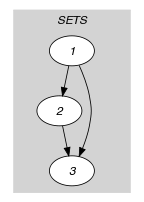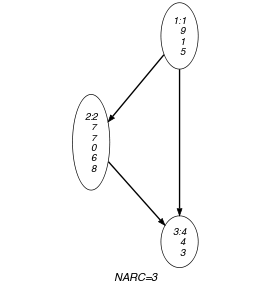## 5.206. k_disjoint

Origin
Constraint

$𝚔_\mathrm{𝚍𝚒𝚜𝚓𝚘𝚒𝚗𝚝}\left(\mathrm{𝚂𝙴𝚃𝚂}\right)$

Type
 $\mathrm{𝚅𝙰𝚁𝙸𝙰𝙱𝙻𝙴𝚂}$ $\mathrm{𝚌𝚘𝚕𝚕𝚎𝚌𝚝𝚒𝚘𝚗}\left(\mathrm{𝚟𝚊𝚛}-\mathrm{𝚍𝚟𝚊𝚛}\right)$
Argument
 $\mathrm{𝚂𝙴𝚃𝚂}$ $\mathrm{𝚌𝚘𝚕𝚕𝚎𝚌𝚝𝚒𝚘𝚗}\left(\mathrm{𝚜𝚎𝚝}-\mathrm{𝚅𝙰𝚁𝙸𝙰𝙱𝙻𝙴𝚂}\right)$
Restrictions
 $\mathrm{𝚛𝚎𝚚𝚞𝚒𝚛𝚎𝚍}$$\left(\mathrm{𝚅𝙰𝚁𝙸𝙰𝙱𝙻𝙴𝚂},\mathrm{𝚟𝚊𝚛}\right)$ $|\mathrm{𝚅𝙰𝚁𝙸𝙰𝙱𝙻𝙴𝚂}|\ge 1$ $\mathrm{𝚛𝚎𝚚𝚞𝚒𝚛𝚎𝚍}$$\left(\mathrm{𝚂𝙴𝚃𝚂},\mathrm{𝚜𝚎𝚝}\right)$ $|\mathrm{𝚂𝙴𝚃𝚂}|>1$
Purpose

Given $|\mathrm{𝚂𝙴𝚃𝚂}|$ sets of domain variables, the $𝚔_\mathrm{𝚍𝚒𝚜𝚓𝚘𝚒𝚗𝚝}$ constraint forces that no value is assigned to more than one set.

Example
$\left(〈\mathrm{𝚜𝚎𝚝}-〈1,9,1,5〉,\mathrm{𝚜𝚎𝚝}-〈2,7,7,0,6,8〉,\mathrm{𝚜𝚎𝚝}-〈4,4,3〉〉\right)$

The $𝚔_\mathrm{𝚍𝚒𝚜𝚓𝚘𝚒𝚗𝚝}$ constraint holds since:

• The set of values $\left\{1,5,9\right\}$ and $\left\{0,2,6,7,8\right\}$ respectively assigned to the variables of the first and second collections have an empty intersection.

• The set of values $\left\{1,5,9\right\}$ and $\left\{3,4\right\}$ respectively assigned to the variables of the first and third collections have an empty intersection.

• The set of values $\left\{0,2,6,7,8\right\}$ and $\left\{3,4\right\}$ respectively assigned to the variables of the second and third collections have an empty intersection.

Typical
$|\mathrm{𝚅𝙰𝚁𝙸𝙰𝙱𝙻𝙴𝚂}|>1$
Symmetries
• Items of $\mathrm{𝚂𝙴𝚃𝚂}$ are permutable.

• Items of $\mathrm{𝚂𝙴𝚃𝚂}.\mathrm{𝚜𝚎𝚝}$ are permutable.

• An occurrence of a value of $\mathrm{𝚅𝙰𝚁𝙸𝙰𝙱𝙻𝙴𝚂}.\mathrm{𝚟𝚊𝚛}$ can be replaced by any value of $\mathrm{𝚅𝙰𝚁𝙸𝙰𝙱𝙻𝙴𝚂}.\mathrm{𝚟𝚊𝚛}$.

• All occurrences of two distinct values of $\mathrm{𝚂𝙴𝚃𝚂}.\mathrm{𝚜𝚎𝚝}.\mathrm{𝚟𝚊𝚛}$ can be swapped; all occurrences of a value of $\mathrm{𝚂𝙴𝚃𝚂}.\mathrm{𝚜𝚎𝚝}.\mathrm{𝚟𝚊𝚛}$ can be renamed to any unused value.

Arg. properties

Contractible wrt. $\mathrm{𝚂𝙴𝚃𝚂}$.

Keywords
Arc input(s)

$\mathrm{𝚂𝙴𝚃𝚂}$

Arc generator
$\mathrm{𝐶𝐿𝐼𝑄𝑈𝐸}$$\left(<\right)↦\mathrm{𝚌𝚘𝚕𝚕𝚎𝚌𝚝𝚒𝚘𝚗}\left(\mathrm{𝚜𝚎𝚝}\mathtt{1},\mathrm{𝚜𝚎𝚝}\mathtt{2}\right)$

Arc arity
Arc constraint(s)
$\mathrm{𝚍𝚒𝚜𝚓𝚘𝚒𝚗𝚝}$$\left(\mathrm{𝚜𝚎𝚝}\mathtt{1}.\mathrm{𝚜𝚎𝚝},\mathrm{𝚜𝚎𝚝}\mathtt{2}.\mathrm{𝚜𝚎𝚝}\right)$
Graph property(ies)
$\mathrm{𝐍𝐀𝐑𝐂}$$=|\mathrm{𝚂𝙴𝚃𝚂}|*\left(|\mathrm{𝚂𝙴𝚃𝚂}|-1\right)/2$

Graph model

Parts (A) and (B) of Figure 5.206.1 respectively show the initial and final graph associated with the Example slot. To each vertex corresponds a collection of variables, while to each arc corresponds a $\mathrm{𝚍𝚒𝚜𝚓𝚘𝚒𝚗𝚝}$ constraint.

##### Figure 5.206.1. Initial and final graph of the $𝚔_\mathrm{𝚍𝚒𝚜𝚓𝚘𝚒𝚗𝚝}$ constraint(a) (b)